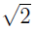# こつこつアルゴリズム

Pell方程式

Secant法
Steffensen法
Newton法

こつこつアルゴリズム

## 平方根とこつこつアルゴリズム

### C:\BASICprograms\spigotDo.BAS

```   1: ! Calculation of square root of 2 by spigot algorithm
2: ! sqrt(2) = 7/5(1-1/50)^(-1/2)
3: !         = 0 + 7/5(1+1/100(1+3/200(1+5/300(1+...))))
4: ! reference
5: ! D.-K.Do, Spigot algorithm and root computing,
6: ! Reliable Computing, vol.7 (2001), 247-273
7: !
8: ! a, b, c and result are arrays; n and i are natural numbers.  The
9: ! original FNE is given by a, b, c and n; c is also used for holding
10: ! the remain nested expressions during computing process; i governs
11: ! the required accuracy; the result number is held in result.
12:
13: INPUT PROMPT "Digits to be calculated = ":L
14: LET n = INT(0.589 * (L + 1) + 1)
15: LET i = L + 3
16:
17: DIM a(1 TO n)
18: DIM b(1 TO n)
19: DIM c(0 TO n)
20: DIM result(0 TO i)
21:
22: LET c(0) = 0
23: LET a(1) = 7
24: LET b(1) = 5
25: LET c(1) = 1
26: FOR j = 2 TO n
27:    LET a(j) = 2 * j - 3
28:    LET b(j) = 100 * (j - 1)
29:    LET c(j) = 1
30: NEXT j
31: FOR j = 0 TO i
32:    LET result(j) = 0
33: NEXT j
34:
35: ! Compute the regular form of original FNE.  This step can be
36: ! omitted, however, is useful for avoiding arithmetic overflow.
37: LET carry = 0
38: FOR j = n TO 1 STEP -1
39:    LET help = c(j) + carry
40:    LET carry = INT(help / 10)
41:    LET c(j) = help - 10 * carry
42: NEXT j
43: LET c(0) = c(0) + carry
44:
45: ! Extract i non-regular digits
46: FOR j = 1 TO i
47:    LET carry = 0
48:    ! Multiply the j-th remain nested expression held in c with
49:    ! 10, compute its regular form and write hte result in c.
50:    FOR k = n TO 1 STEP -1
51:       LET help = 10 * c(k) + carry
52:       LET carry = INT(help / b(k))
53:       LET c(k) = help - b(k) * carry
54:       LET carry = carry * a(k)
55:    NEXT k
56:    ! extract the free coefficient
57:    LET result(j) = carry
58: NEXT j
59: ! Compute the regular form to make the final result.
60: LET carry = 0
61: FOR k = i TO 1 STEP -1
62:    LET help = result(k) + carry
63:    LET carry = INT(help / 10)
64:    LET result(k) = help - 10 * carry
65: NEXT k
66: LET result(0) = result(0) + carry
67:
68: ! Print out the final result
69: PRINT USING "#.":result(0);
70: FOR k = 1 TO L
71:    PRINT USING "#": result(k);
72: NEXT k
73: PRINT
74: END
```

ここで示したプログラムでは、に限らず、次の形に表される級数について計算が可能です。

ここから先、工事中です。近日公開予定。